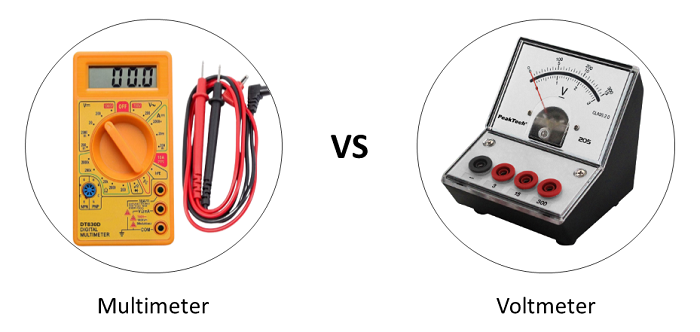# Difference between Multimeter and Voltmeter

Multimeter and Voltmeter are handheld measuring devices. Voltmeters are used when one needs to measure only voltage. Multimeters, in contrast, can be used to measure multiple electrical quantities such as current, voltage, resistance, etc.

Go through this article to find out the features of multimeters and voltmeters and how they differ from each other.## What is a Multimeter?

Multimeter, as the name implies, is a combination of different measuring instruments in a single unit. It can measure several electrical quantities such as current, voltage, resistance, etc. An extended version of multimeter can also measure other electrical properties like transistors gain, diode forward voltage, capacitance, etc.

A typical multimeter detects the fault in the electric circuit and also gives the values of electrical parameter in the standard unit.

A basic multimeter is a combination of a DC voltmeter, AC voltmeter, DC ammeter, AC ammeter and ohmmeter. The multimeter is one of the important measuring instrument which is preferred by electricians for troubleshooting the problems in electrical appliances, motors, circuits, etc.

### Types of Multimeter

Based on the design and method of measurement and display, the multimeters are classified into two types as −

• Analog Multimeter
• Digital Multimeter

An analog multimeter is a type of multimeter based around the use of a moving coil meter. It consists of a display of analog form that uses the deflection of a pointer to indicate the level of the measurement. The analog multimeters are mainly used to measure electrical quantities like voltage, current, resistance, frequency and power, etc.

A digital multimeter (DMM) is a measuring device which can also be used to measure electrical quantities such as voltage, current and resistance. In a digital multimeter, the measured value of the quantity is displayed on a digital screen.

## What is a Voltmeter?

An electrical measuring instrument which is used for measuring voltage (or electric potential difference) between two points in an electrical circuit is known as voltmeter. It is also known as voltage meter.

In order to measure the voltage across a device, the voltmeter is connected in parallel to the devices. It is connected in parallel so that same voltage drop occurs across both device and voltmeter.

A voltmeter has a very high internal resistance so there is no current flow through the voltmeter, this allows the voltmeter to record correct voltage readings.

### Types of Voltmeter

Depending on the method of measurement and display, voltmeters are of two types as −

• Analog voltmeter
• Digital voltmeter

An analog voltmeter is a type of voltmeter which gives readings by deflecting a pointer on a scale. While, a digital voltmeter (DVM) gives the readings of measurement on a seven-segment display.

## Difference between Multimeter and Voltmeter

The following table highlights the key differences between a multimeter and a voltmeter −

ParameterMultimeterVoltmeter
DefinitionA multimeter is a multi-purpose measuring device which can directly measure various electrical quantities such as voltage, current and resistance, etc.A voltmeter is an electrical measuring instrument which can measure only voltage (or potential difference) between two points in an electric circuit.
FunctionA multimeter combines the functions of various meters such as voltmeter, ammeter and ohmmeter, etc. in a single unit.The function of a voltmeter is only to measure the voltage between two points in an electric circuit.
Circuit connectionThe connection of the multimeter in an electric circuit depends upon the quantity to be measured. For example, to measure voltage, it is connected in parallel to the device, and to measure circuit current, is connected in series.The voltmeter is always connected in parallel with device whose voltage is to be measured.
Internal resistanceThe internal resistance of the multimeter is variable depending upon the function of the meter.The voltmeter has a high internal resistance so that no current flows through it.
AC and DC MeasurementA single multimeter can be used to measure both AC and DC quantities.For AC and DC voltage measurement, separate voltmeters are required.
ConstructionAs a multimeter combines various functions in a single unit, thus its construction is complex.A voltmeter has only one circuit for measuring the voltage, therefore, it is relatively simple in construction.
Need power suppledA multimeter (digital multimeter) requires a power supply to function.A voltmeter does not require device power supply to work.
SizeA multimeter is relatively bigger in size.A voltmeter is smaller in size.
VersatilityA multimeter is a versatile instrument because it can be used to measure various electrical properties.It is not a versatile instrument because it is only able measure potential difference between two points.
ScaleA multimeter has its scale calibrated for measuring various electrical quantities like ampere, volts, ohms, etc.A voltmeter has a scale which is calibrated to measure the voltage in volts, kV, etc.
CostA multimeter is relatively expensive as it offers wide range of features.A voltmeter is cheaper than a multimeter as it limited to measure only voltage.
SubstitutionA multimeter can be used as a voltmeter.Voltmeter cannot substitute a multimeter.
Testing of diode and transistorsA multimeter can be used to test diodes and transistors.A voltmeter cannot be used to test diodes and transistors.
ApplicationA multimeter is used for the measurement of voltage, current, resistance, capacitance, continuity, diode forward voltage, etc.A voltmeter is used to measure only voltage in the circuit. Thus, its area of application is comparatively less.

## Conclusion

To conclude, the difference between a multimeter and a voltmeter is quite straightforward. If we need to measure only voltage in an electric circuit, then we use a voltmeter; however if we need to measure other properties like current, resistance, etc. along with the voltage, then we need to use a multimeter.